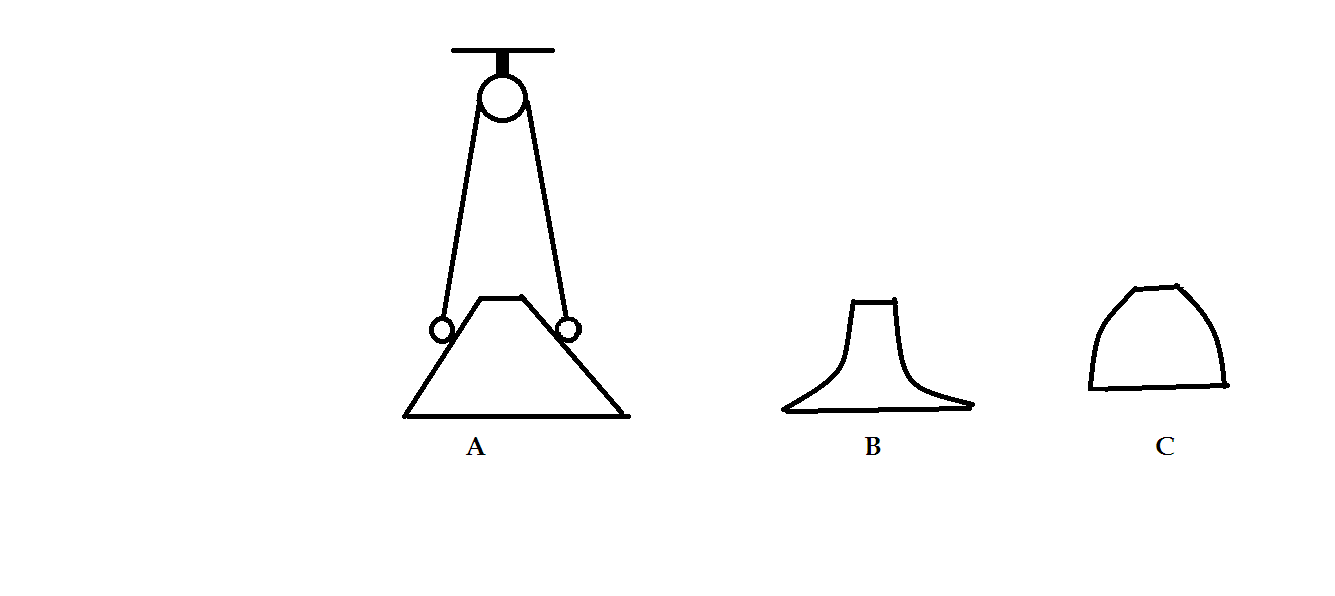# Vector analysis

Draw a circle in the xy plane .At a few representative points draw the vector v tangent to the circle ,pointing in the clockwise direction.By comparing adjacent vectors,determine the sign of dv sub x/dy and dv sub y/dx .According to cartesian construct of curl that is cross product expansion.What is the direction curl of vector v?Explain how it illustrates the geometrical interpretation of curl.(Here d/dx and d/dy stands for partial derivatives and sub x and y means the x and y component of vector v )Note by Rishi Tiwari
10 months, 4 weeks ago

MarkdownAppears as
*italics* or _italics_ italics
**bold** or __bold__ bold
- bulleted- list
• bulleted
• list
1. numbered2. list
1. numbered
2. list
Note: you must add a full line of space before and after lists for them to show up correctly
paragraph 1paragraph 2

paragraph 1

paragraph 2

[example link](https://brilliant.org)example link
> This is a quote
This is a quote
    # I indented these lines
# 4 spaces, and now they show
# up as a code block.

print "hello world"
# I indented these lines
# 4 spaces, and now they show
# up as a code block.

print "hello world"
MathAppears as
Remember to wrap math in $ ... $ or $ ... $ to ensure proper formatting.
2 \times 3 $2 \times 3$
2^{34} $2^{34}$
a_{i-1} $a_{i-1}$
\frac{2}{3} $\frac{2}{3}$
\sqrt{2} $\sqrt{2}$
\sum_{i=1}^3 $\sum_{i=1}^3$
\sin \theta $\sin \theta$
\boxed{123} $\boxed{123}$

Sort by:

@Steven Chase Help me with this new problem about equilibria of pulleys

- 10 months, 2 weeks ago

@Steven Chase @Josh Silverman As shown in the following fig.in the given three arrangements A,B and C where pulleys are ideal,strings are light and inextensible,balls attached at the ends of strings are identical and surfaces where the balls rest are frictionless .Identify the nature of equilibria (i.e stable,unstable or neutral) of the arrangements . @Steven Chase @Josh Silverman Now in the figure shown we have to assume that pulley like in A arrangement is also in B and C arrangement but instead of inclined surfaces concavity in B and convexity in C is introduced as it was super hard for me to draw such figures with mouse .PLS give detailed solutions- 10 months, 2 weeks ago

@Steven Chase and @Josh Silverman.Help me with this As shown in the following fig.in the given three arrangements A,B and C where pulleys are ideal,strings are light and inextensible,balls attached at the ends of strings are identical and surfaces where the balls rest are frictionless .Identify the nature of equilibria (i.e stable,unstable or neutral) of the arrangements . @Steven Chase @Josh Silverman Now in the figure shown we have to assume that pulley like in A arrangement is also in B and C arrangement but instead of inclined surfaces concavity in B and convexity in C is introduced as it was super hard for me to draw such figures with mouse .PLS give detailed solutions- 10 months, 2 weeks ago

Thanks a lot can you recommend me something on books ,videos,websites,problems regarding the preparation of Indian National Physics Olympiad.

- 10 months, 3 weeks ago

I haven't been involved with those Olympiads, but there are many on this site who have. I'm sure they could give you some suggestions.

- 10 months, 3 weeks ago

As shown in the following fig.in the given three arrangements A,B and C where pulleys are ideal,strings are light and inextensible,balls attached at the ends of strings are identical and surfaces where the balls rest are frictionless .Identify the nature of equilibria (i.e stable,unstable or neutral) of the arrangements . @Steven Chase @Josh Silverman Now in the figure shown we have to assume that pulley like in A arrangement is also in B and C arrangement but instead of inclined surfaces concavity in B and convexity in C is introduced as it was super hard for me to draw such figures with mouse .PLS give detailed solutions- 10 months, 2 weeks ago

Define some terms on the unit circle (assuming the tangent vector $\vec{v}$ is clockwise).

$\large{x = cos \theta \\ y = sin \theta \\ z = 0 \\ v_x = sin \theta = y \\ v_y = -cos \theta = -x \\ v_z = 0}$

Take partial derivatives:

$\large{\frac{\partial{v_x}}{\partial{y}} = 1 \\ \frac{\partial{v_y}}{\partial{x}} = -1}$

Suppose the curl of $\vec{v}$ is $\vec{C}$:

$\large{C_x = \frac{\partial{v_z}}{\partial{y}} - \frac{\partial{v_y}}{\partial{z}} = 0 \\ C_y = -\frac{\partial{v_z}}{\partial{x}} + \frac{\partial{v_x}}{\partial{z}} = 0 \\ C_z = \frac{\partial{v_y}}{\partial{x}} - \frac{\partial{v_x}}{\partial{y}} = -2}$

The curl of $\vec{v}$ is therefore a vector in the $- z$ direction, which agrees with the well-known right-hand rule.

- 10 months, 3 weeks ago

pls help me with this problem ASAP https://brilliant.org/discussions/thread/potential-energy/

- 8 months, 3 weeks ago

Thanks a lot you always help me I did the same way by introducing same parameters in the circle it assures that my attempt was right.As author has directly written the vector v even making v sub z non zero but that makes sense because choice of v sub z should be in a manner that C sub x and C sub y should be zero that is derivatives should cancel out to zero in order to make consistency with right hand rule

- 10 months, 3 weeks ago

- 10 months, 4 weeks ago

Help asap

- 10 months, 4 weeks ago

@Steven Chase

- 10 months, 4 weeks ago

@Josh Silverman @Steven Chase

- 10 months, 4 weeks ago